In this vignette we demonstrate clustering of 3rd complementary determining region sequence (CDR3) and V-J gene identity of mouse T cells, ways to visualize and explore clusters that are expanded, pairing of alpha-beta clusters, tests of differential CDR3 usage, and permutation tests for overall clonal properties.

library(CellaRepertorium)
library(dplyr)
#>
#> Attaching package: 'dplyr'
#> The following objects are masked from 'package:stats':
#>
#>     filter, lag
#> The following objects are masked from 'package:base':
#>
#>     intersect, setdiff, setequal, union
library(ggplot2)
library(tidyr)
library(stringr)
library(purrr)

We begin with a data.frame of concatenated contig files (‘all_contig_annotations.csv’), output from the Cellranger VDJ pipeline.

data(contigs_qc)
MIN_CDR3_AA = 6

cdb = ContigCellDB_10XVDJ(contigs_qc, contig_pk = c('barcode', 'pop', 'sample', 'contig_id'), cell_pk = c('barcode', 'pop', 'sample'))
cdb
#> ContigCellDB of 1508 contigs; 832 cells; and 0 clusters.
#> Contigs keyed by barcode, pop, sample, contig_id; cells keyed by barcode, pop, sample.

Initially we start with 832 cells and 1508 contigs. We keep contigs that are

• full - length
• productive
• high-confidence
• only from T cells
• and with CDR3 sufficiently long.

cdb$contig_tbl = dplyr::filter(cdb$contig_tbl, full_length, productive == 'True', high_confidence, chain != 'Multi', str_length(cdr3) > MIN_CDR3_AA) %>% mutate( fancy_name = fancy_name_contigs(., str_c(pop, '_', sample)))

After filtering, there are 832 cells and 1496 contigs.

## Clustering contigs by sequence characteristics

As a first step to define clonotypes, we will first find equivalence classes of CDR3 sequences with the program CD-HIT. In this case, we use the translated amino acid residues, but often one might prefer to use the DNA sequences, by setting the sequence_key accordingly and type = 'DNA'. Additionally, a higher identity threshold might be appropriate (see below).

aa80 = cdhit_ccdb(cdb, sequence_key = 'cdr3', type = 'AA', cluster_pk = 'aa80',
identity = .8, min_length = 5, G = 1)
aa80 = fine_clustering(aa80, sequence_key = 'cdr3', type = 'AA', keep_clustering_details = TRUE)
#> Calculating intradistances on 988 clusters.
#> Summarizing

This partitions sequences into sets with >80% mutual similarity in the amino acid sequence, adds some additional information about the clustering, and returns it as a ContigCellDB object named aa80. The primary key for the clusters is aa80. The min_length can be set somewhat smaller, but there is a lower limit for the cdhit algorithm. G=1, the default, specifies a global alignment. This is almost always what is desired, but local alignment is available if G=0.

head(aa80$cluster_tbl) #> # A tibble: 6 × 4 #> aa80 avg_distance fc n_cluster #> <dbl> <dbl> <list> <int> #> 1 1 0 <named list > 1 #> 2 2 0 <named list > 1 #> 3 3 0 <named list > 2 #> 4 4 0 <named list > 1 #> 5 5 0 <named list > 1 #> 6 6 0 <named list > 1 head(aa80$contig_tbl) %>% select(contig_id, aa80, is_medoid, d(medoid))
#> # A tibble: 6 × 4
#>   contig_id                    aa80 is_medoid d(medoid)
#>   <chr>                       <dbl> <lgl>           <dbl>
#> 1 ATCTACTCAGTATGCT-1_contig_3     1 TRUE                0
#> 2 ACTGTCCTCAATCACG-1_contig_3     2 TRUE                0
#> 3 CACCTTGTCCAATGGT-1_contig_2     3 TRUE                0
#> 4 CACCTTGTCCAATGGT-1_contig_2     3 FALSE               0
#> 5 CGGACGTGTTCATGGT-1_contig_1     4 TRUE                0
#> 6 CTGCTGTTCCCTAATT-1_contig_4     5 TRUE                0

The cluster_tbl lists the 988 80% identity groups found, including the number of contigs in the cluster, and the average distance between elements in the group. In the contig_tbl, there are two columns specifying if the contig is_medoid, that is, is the most representative element of the set and the distance to the medoid element d(medoid).

cluster_plot(aa80)
#> stat_bin() using bins = 30. Pick better value with binwidth.
#> stat_bin() using bins = 30. Pick better value with binwidth.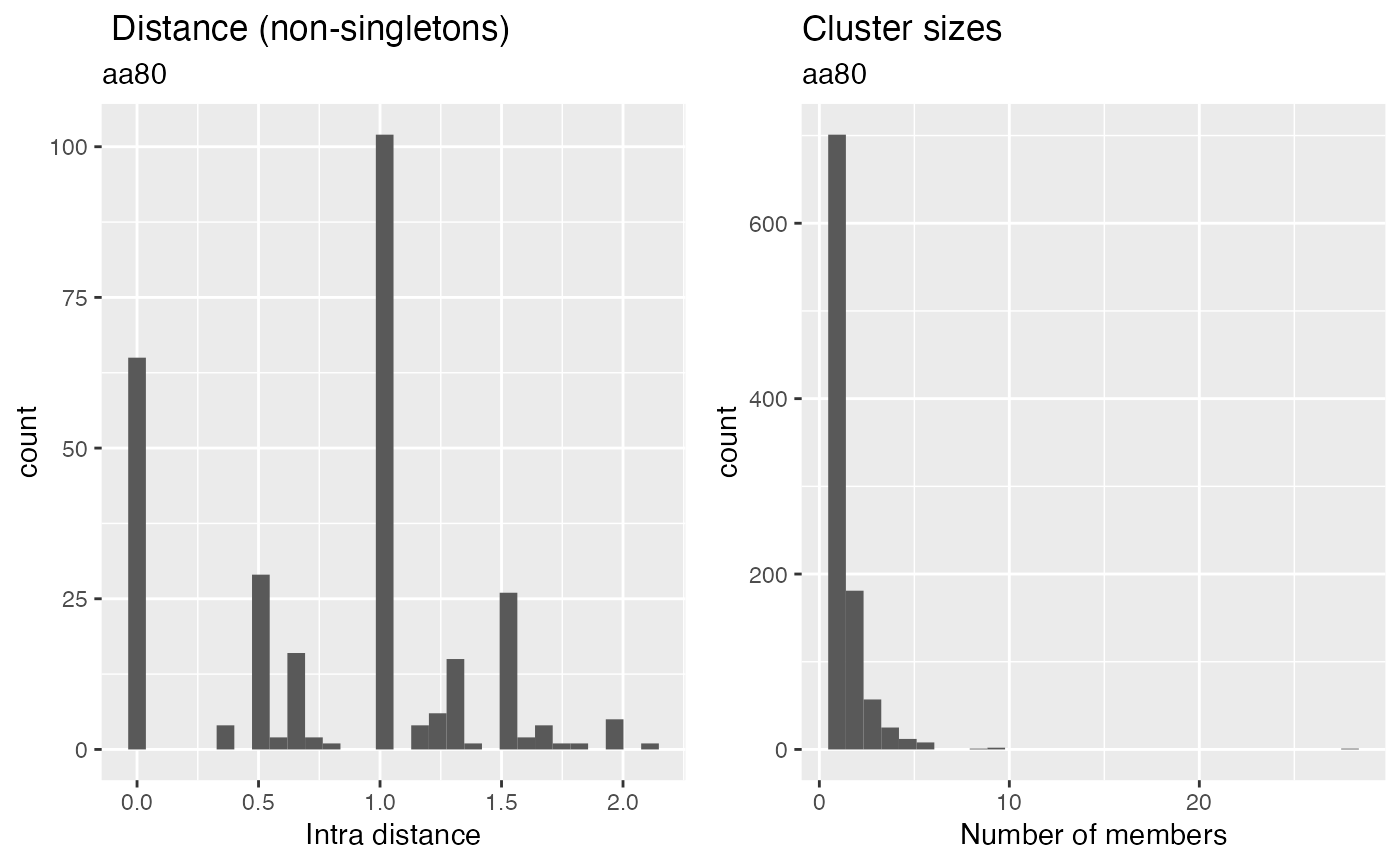### Cluster CDR3 DNA sequences

cdb = cdhit_ccdb(cdb, 'cdr3_nt', type = 'DNA', cluster_pk = 'DNA97', identity = .965, min_length = MIN_CDR3_AA*3-1, G = 1)
cdb = fine_clustering(cdb, sequence_key = 'cdr3_nt', type = 'DNA')
#> Calculating intradistances on 1342 clusters.
#> Summarizing

cluster_plot(cdb)
#> stat_bin() using bins = 30. Pick better value with binwidth.
#> stat_bin() using bins = 30. Pick better value with binwidth.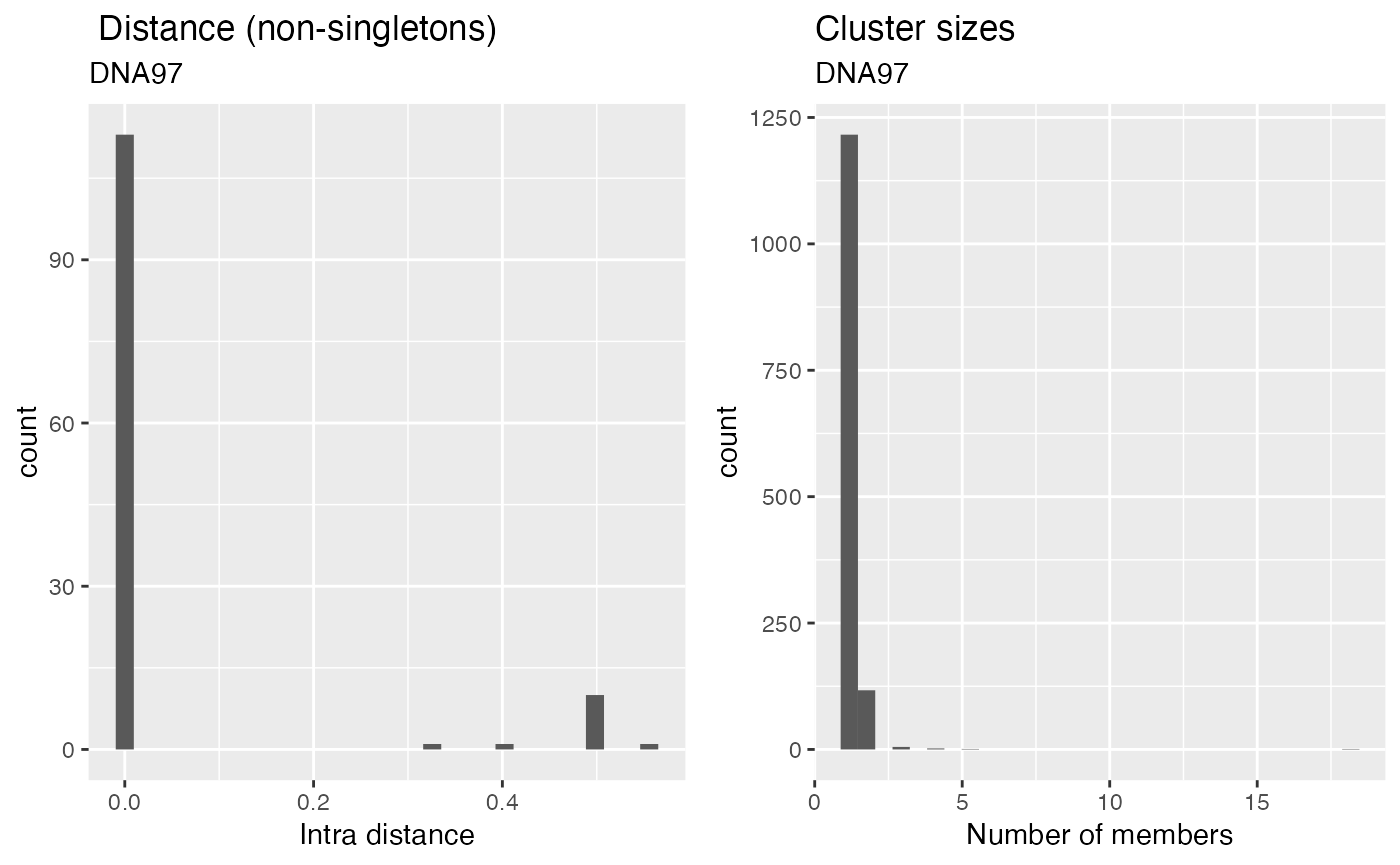We can also cluster by DNA identity.

### Cluster by V-J identity

germline_cluster = cluster_germline(cdb, segment_keys = c('v_gene', 'j_gene', 'chain'), cluster_pk = 'segment_idx')
#> Warning in replace_cluster_tbl(ccdb, cluster_tbl, cl_con_tbl, cluster_pk =
#> cluster_pk): Replacing cluster_tbl with DNA97.

We can cluster by any other feature of the contigs. Here we cluster each contig based on the chain and V-J genes. This gives us the set of observed V-J pairings:

germline_cluster = fine_clustering(germline_cluster, sequence_key = 'cdr3_nt', type = 'DNA')
#> Calculating intradistances on 700 clusters.
#> Summarizing
#> Warning in left_join_warn(d_medoid, contig_tbl, by = ccdb$contig_pk, overwrite = #> TRUE): Overwriting fields d(medoid), is_medoid in table contig_tbl filter_cdb(germline_cluster, chain == 'TRB') %>% plot_cluster_factors(factors = c('v_gene','j_gene'), statistic = 'contigs', type = 'heatmap')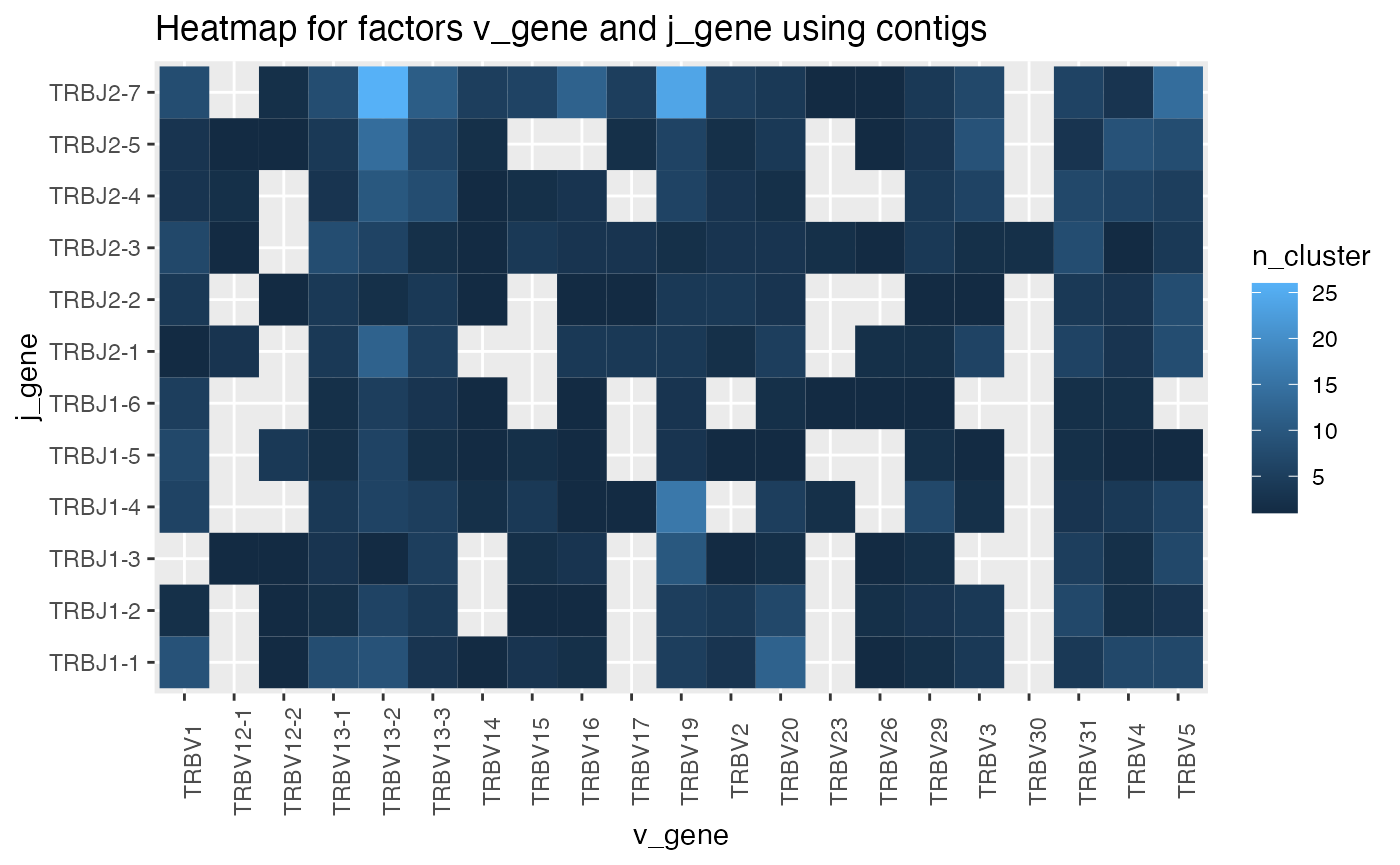Number of pairs. The pearson residual (showing the difference from expected counts given marginals) is probably more informative, set statistic = 'residual' for this. ggplot(germline_cluster$cluster_tbl %>% filter(chain == 'TRB'), aes(x = v_gene, y = j_gene, fill = avg_distance)) + geom_tile() + theme(axis.text.x = element_text(angle = 90))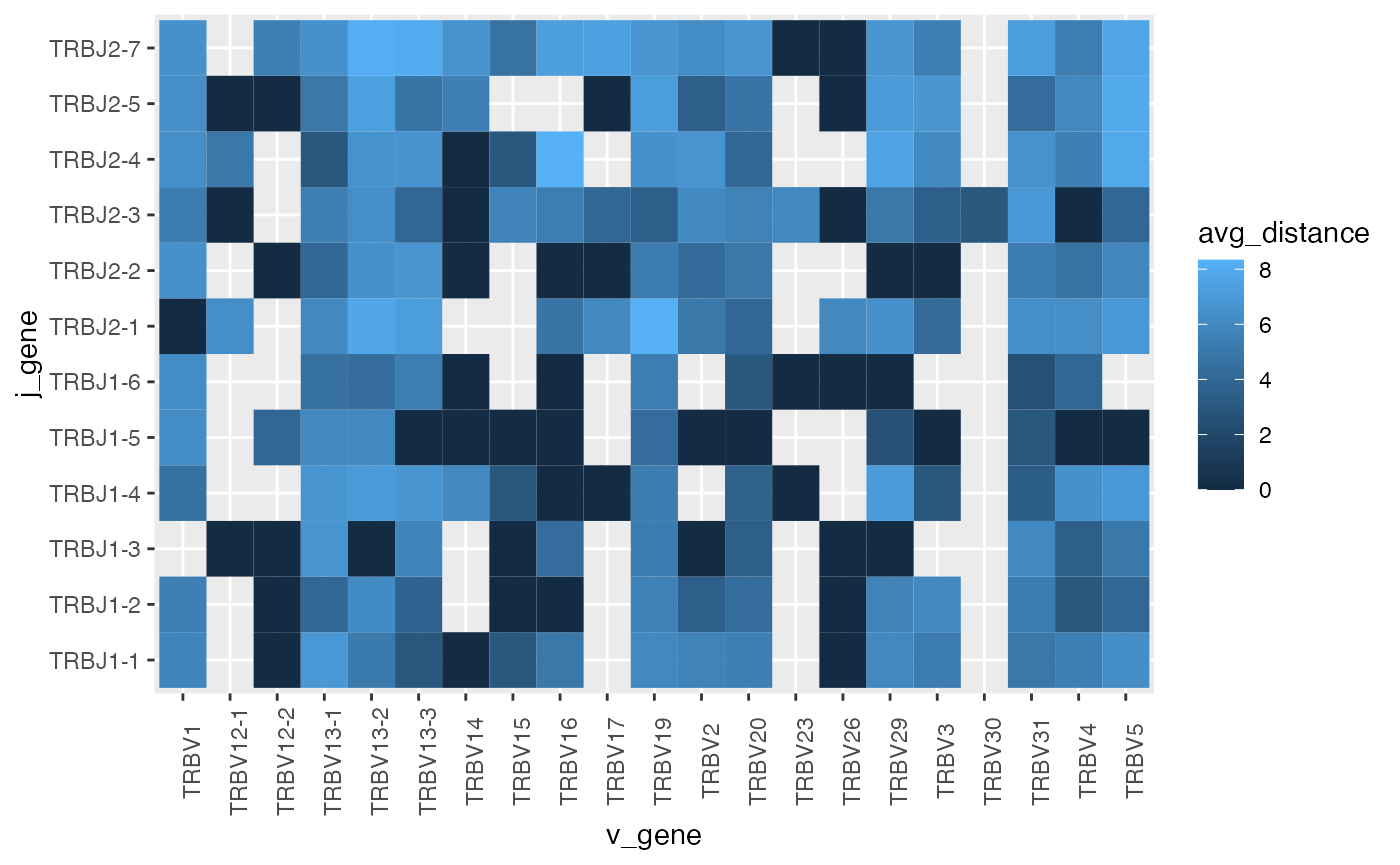Average Levenshtein distance of CDR3 within each pair. This might be turned into a z-score by fitting a weighted linear model with sum-to-zero contrasts and returning the studentized residuals. This could determine if a pairing has an unexpected small, or large, within cluster distance.

### Expanded clusters

Next, we will examine the clusters that are found in many contigs. First we will get a canonical contig to represent each cluster. This will be the medoid contig, by default.

aa80 = canonicalize_cluster(aa80, representative = 'cdr3', contig_fields = c('cdr3', 'cdr3_nt', 'chain', 'v_gene', 'd_gene', 'j_gene'))
#> Filtering contig_tbl by is_medoid, override by setting contig_filter_args == TRUE

aa80 now includes the fields listed in contig_fields in the cluster_tbl, using the values found in the medoid contig.

MIN_OLIGO = 7
oligo_clusters = filter(aa80$cluster_tbl, n_cluster >= MIN_OLIGO) oligo_contigs = aa80 oligo_contigs$contig_tbl = semi_join(oligo_contigs$contig_tbl, oligo_clusters, by = 'aa80') oligo_contigs #> ContigCellDB of 54 contigs; 832 cells; and 4 clusters. #> Contigs keyed by barcode, pop, sample, contig_id; cells keyed by barcode, pop, sample. Get contigs/cells/clusters found at least 7 times (across contigs). Note that replacing contig_tbl with the subset selected with the semi_join also automatically subsetted the cell_tbl and cluster_tbl. oligo_clusters = oligo_contigs$contig_tbl %>% group_by(aa80) %>% summarize(n subjects observed = length(unique(sample))) %>% left_join(oligo_clusters)
#> Joining, by = "aa80"

knitr::kable(oligo_clusters %>% select(aa80:cdr3, chain:j_gene, avg_distance, n_cluster))
aa80 n subjects observed cdr3 chain v_gene d_gene j_gene avg_distance n_cluster
111 6 CVVGDRGSALGRLHF TRA TRAV11 None TRAJ18 0.6071429 28
172 5 CAVSRASSGSWQLIF TRA TRAV9N-3 None TRAJ22 2.1111111 9
296 6 CAASASSGSWQLIF TRA TRAV14D-2 None TRAJ22 1.5000000 8
808 4 CATGNYAQGLTF TRA TRAV8D-2 None TRAJ26 1.3333333 9

Report some statistics about these expanded clusters, such as how often they are found, how many subjects, etc.

oligo_plot = ggplot(oligo_contigs$contig_tbl, aes(x = representative, fill = chain)) + geom_bar() + coord_flip() + scale_fill_brewer(type = 'qual') + theme_minimal() oligo_plot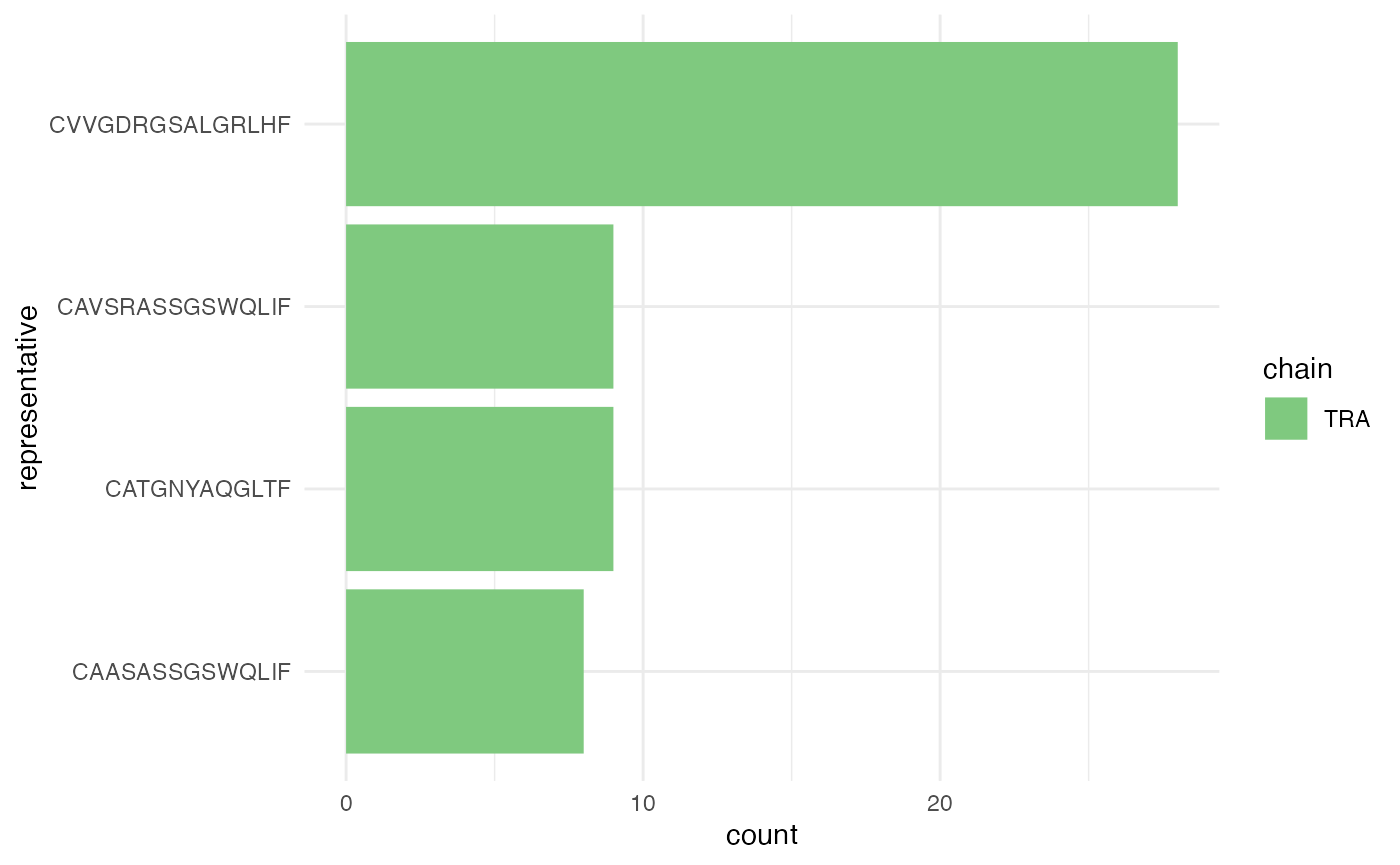These always come from a single chain. oligo_plot + aes(fill = sample) + facet_wrap(~pop)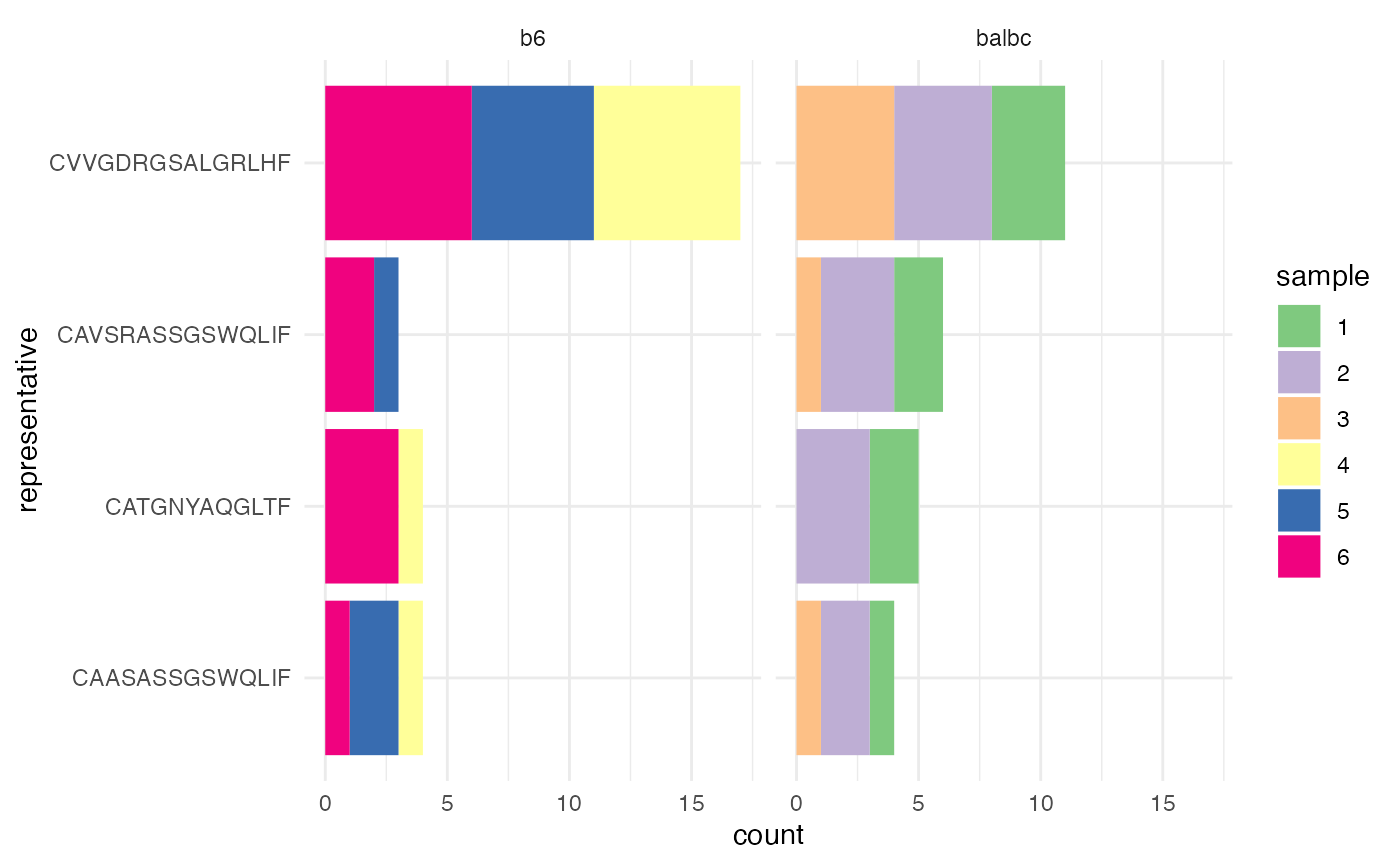But come from multiple populations and samples. ### Some simple phylogenetic relationships By using the within-cluster distances, some rudamentory plots attempting to show phylogenetic associations are possible. (These are most biologically appropriate for B cells that undergo somatic hypermutation.) library(ggdendro) dendro_plot = function(ccdb, idx, method = 'complete'){ h = filter(ccdb$cluster_tbl, !!sym(ccdb$cluster_pk) == idx) %>% pull(fc) %>% .[] quer = filter(ccdb$contig_tbl, !!sym(ccdb$cluster_pk) == idx) hc = hclust(as.dist(h$distance_mat), method = method) %>% dendro_data(type = "rectangle")
hc$labels = cbind(hc$labels, quer)
ggplot(hc$segments, aes(x=x, y=y)) + geom_segment(aes(xend=xend, yend=yend)) + theme_classic() + geom_text(data = hc$labels, aes(color = sample, label = fancy_name), size = 3, angle = 60, hjust =0, vjust = 0) + scale_x_continuous(breaks = NULL) + ylab('AA Distance') + xlab('')
}

to_plot = aa80$cluster_tbl %>% filter(min_rank(-n_cluster) == 1) map(to_plot$aa80, ~ dendro_plot(aa80, .))
#> []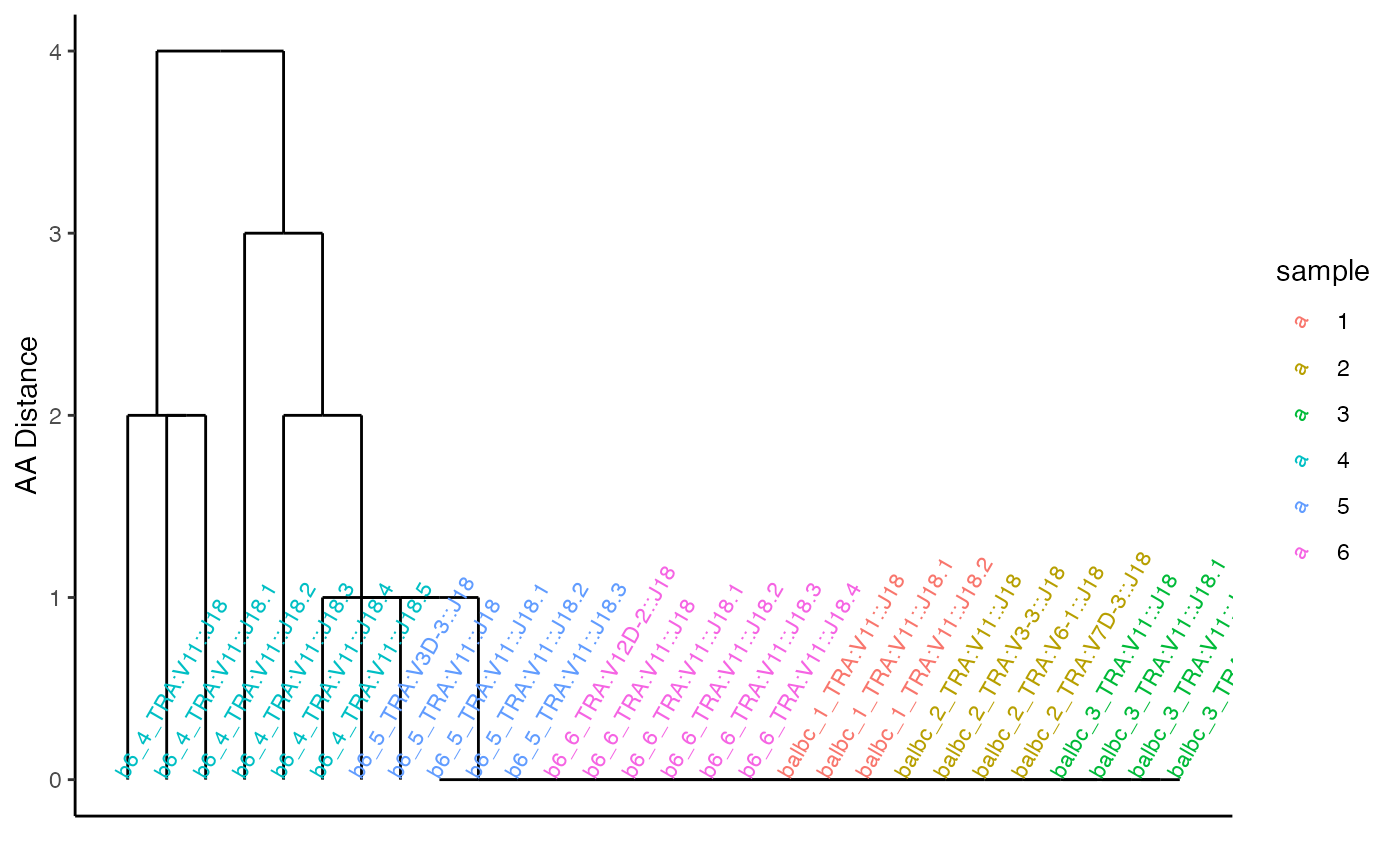A full-blown generative model of clonal generation and selection would be recommended for any actual analysis, but these plots may suffice to get a quick idea of the phylogenetic structure.

### Formal testing for frequency differences

We can test for differential usage of a clone, or cluster with cluster_logistic_test and cluster_test_by. The latter splits the cluster_tbl by field = 'chain', thereby adjusting the number of cell trials included in the “denominator” of the logistic regression. The formula tests for differences between populations, including the sample as a random effect, and only tests clusters that are included in the oligo_clusters set.

mm_out = cluster_test_by(aa80, fields = 'chain', tbl = 'cluster_tbl', formula = ~ pop + (1|sample), filterset = cluster_filterset(white_list = oligo_clusters)) %>%
left_join(oligo_clusters)
#> Fitting mixed logistic models to 4 clusters.
#> boundary (singular) fit: see help('isSingular')
#> boundary (singular) fit: see help('isSingular')
#> boundary (singular) fit: see help('isSingular')
#> Fitting mixed logistic models to 0 clusters.
#> Joining, by = c("chain", "aa80")

mm_out = mutate(mm_out, conf.low = estimate-1.96*std.error,
conf.high = estimate + 1.96*std.error)
mm_outj = filter(ungroup(mm_out), term == 'popbalbc') %>% arrange(desc(representative))

ggplot(mm_outj, aes(x = representative, ymin = conf.low, ymax = conf.high, y = estimate)) + geom_pointrange()  + coord_flip() + theme_minimal() + geom_hline(yintercept = 0, lty = 2) + xlab("Isomorph") + ylab("log odds of isomorph")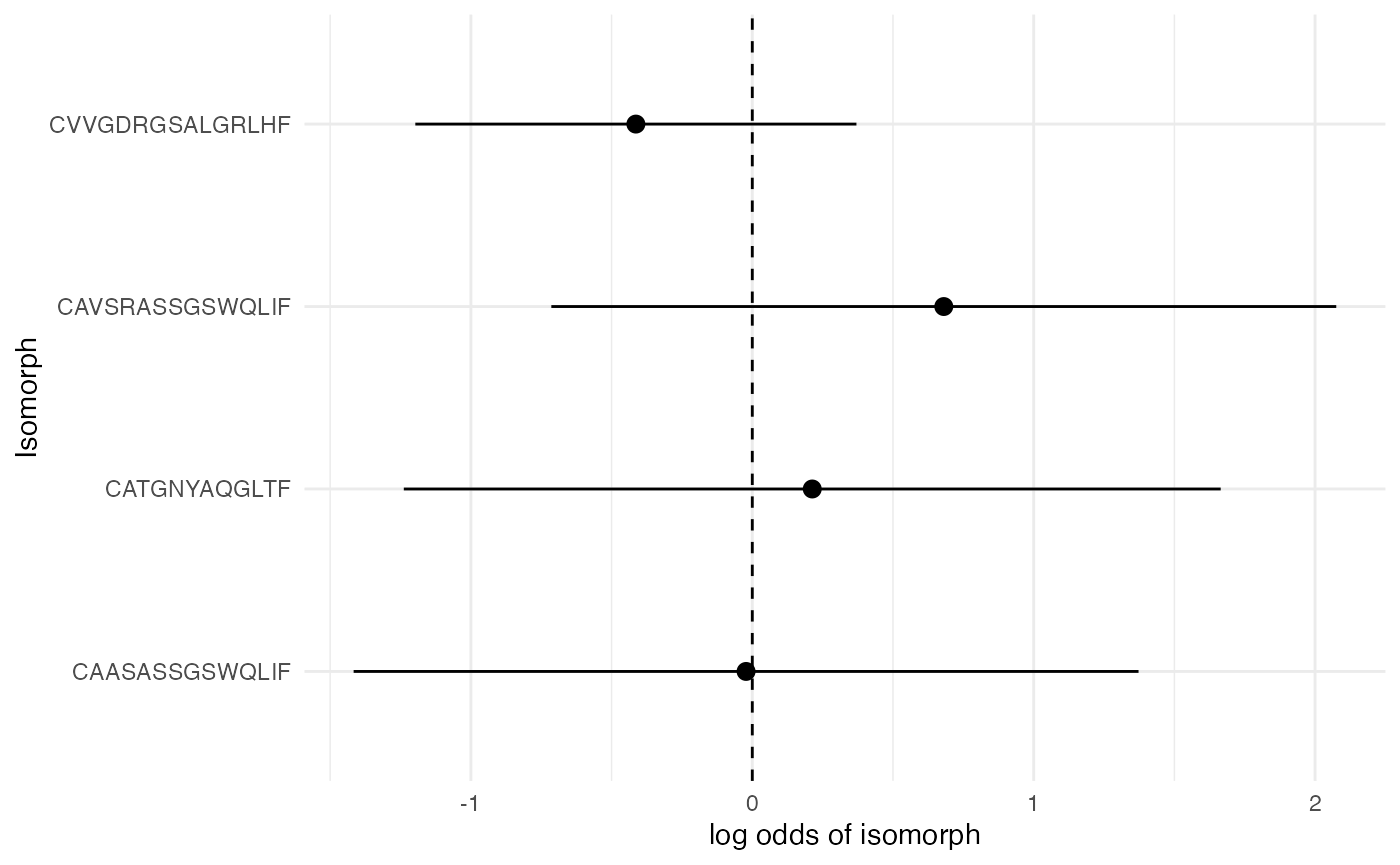We test if the binomial rate of clone expression differs between balbc and b6, for the selected clones. None appear to be different.

### Length of CDR3

aa80$contig_tbl = aa80$contig_tbl %>% mutate(cdr3_length = str_length(cdr3_nt))
ggplot(aa80$contig_tbl, aes(fill = pop, x= cdr3_length)) + geom_histogram(binwidth = 1, mapping = aes(y = ..density..)) + theme_minimal() + scale_fill_brewer(type = 'qual') + facet_grid(sample ~chain) + theme(strip.text.y = element_text(angle = 0)) + coord_cartesian(xlim = c(25, 55))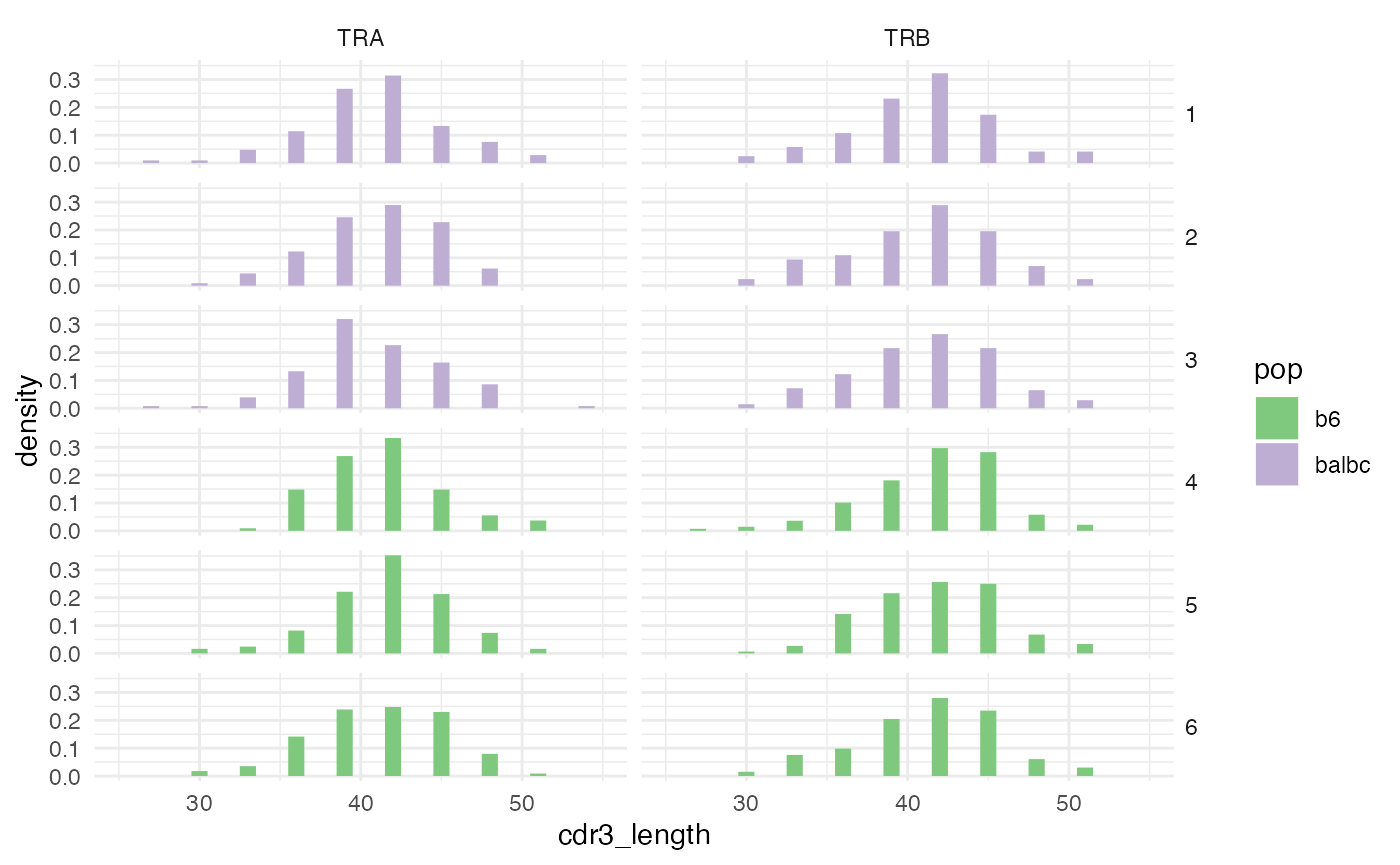Some authors have noted that the length of the CDR3 region can be predictive of T cell differentiation. In our study, there doesn’t appear to be a noticeable difference between BALB/c and C57BL/6J (b6) mice, but if we needed to make sure, an appropriate procedure would be to run a mixed model with a random sample effect (assumed to represent a biological replicate). cdr_len = aa80$contig_tbl %>% group_by(chain) %>% do(broom::tidy(lme4::lmer(cdr3_length ~ pop + (1|sample), data = .), conf.int = TRUE))
#> boundary (singular) fit: see help('isSingular')
#> boundary (singular) fit: see help('isSingular')
ggplot(cdr_len %>% filter(term == 'popbalbc'), aes(x = interaction(chain, term), y = estimate, ymin = conf.low, ymax = conf.high)) +
geom_pointrange() + theme_minimal() + coord_flip() +
ylab('Length(CDR3 Nt)') + xlab('Term/Chain') + geom_hline(yintercept = 0, lty = 2)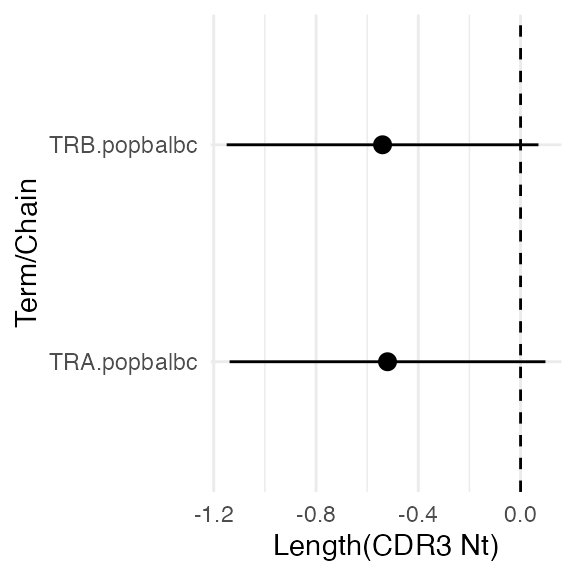We end up with a (harmless) convergence warning about a singular fit. This is expected, because the samples aren’t actually replicates – they are just subsamples drawn for illustrative purposes. The Balbc mice have .5 fewer nucleotides per contig, on average, and this is not significant.

## Clonal pairs

Next, we can examine the pairing between $$\alpha-\beta$$ chains and see if any pairs are found more than once.

aa80$cluster_pk = 'representative' aa80 = rank_prevalence_ccdb(aa80) pairing_list = pairing_tables(aa80, table_order = 2, orphan_level = 1, min_expansion = 3, cluster_keys = c('cdr3', 'representative', 'chain', 'v_gene', 'j_gene', 'avg_distance')) pairing_tables finds all contig combinations of order table_order across cells. Among those combinations that occur at least min_expansion times, the expanded combinations and and any other combinations that shared an expanded combo.  pairs_plt = ggplot(pairing_list$cell_tbl, aes(x = cluster_idx.1_fct, y = cluster_idx.2_fct)) + geom_jitter(aes(color = sample, shape = pop), width = .2, height = .2) + theme_minimal() + xlab('TRB') + ylab('TRA') + theme(axis.text.x = element_text(angle = 45))

pairs_plt = map_axis_labels(pairs_plt, pairing_list$idx1_tbl, pairing_list$idx2_tbl, aes_label  = 'chain')
pairs_plt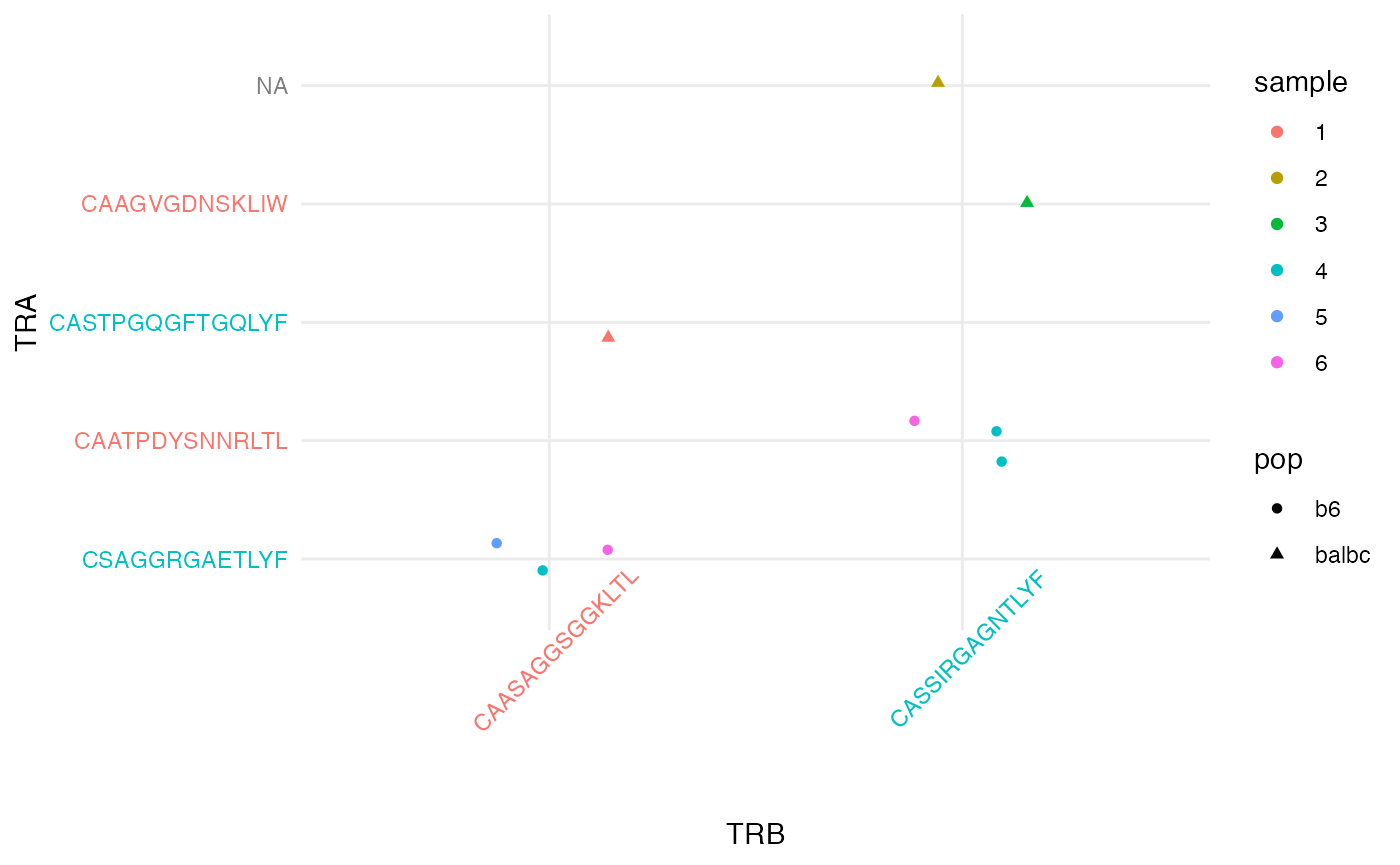### Expanded clones

whitelist = oligo_clusters %>% dplyr::select(cluster_idx.1 = representative) %>% unique()
pairing_list = pairing_tables(aa80, table_order = 2, orphan_level = 1, min_expansion = Inf, cluster_whitelist = whitelist,  cluster_keys = c('cdr3', 'representative', 'chain', 'v_gene', 'j_gene', 'avg_distance'))

pairs_plt = ggplot(pairing_list$cell_tbl, aes(x = cluster_idx.1_fct, y = cluster_idx.2_fct)) + geom_jitter(aes(color = sample, shape = pop), width = .2, height = .2) + theme_minimal() + xlab('TRB') + ylab('TRA') + theme(axis.text.x = element_text(angle = 45)) pairs_plt = map_axis_labels(pairs_plt, pairing_list$idx1_tbl, pairing_list$idx2_tbl, aes_label = 'chain') pairs_plt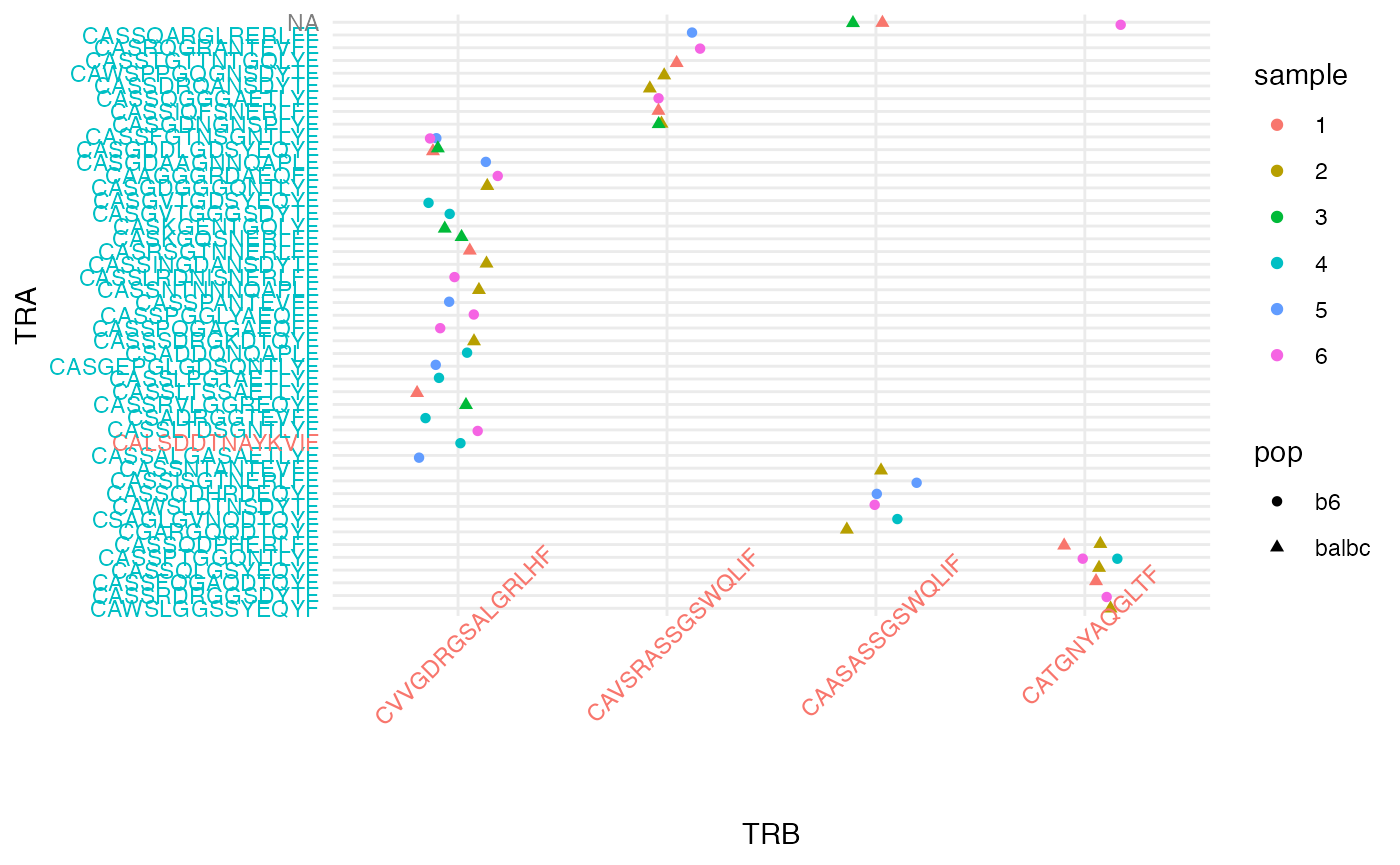By setting min_expansion = Inf, cluster_whitelist = whitelist we can examine any pairings for a set of cluster_idx, in this case the ones that were seen multiple times. Interestingly (and unlike some human samples) the expanded clusters are $$\beta$$-chain, and their $$\alpha$$ chains are sprinkled quite evenly across clusters. ## Permutation tests Permutation tests allow tests of independence between cluster assignments and other cell-level covariates (such as the sample from which the cell was derived). The cluster label is permuted to break the link between cell and cluster, and an arbitrary statistic of both cluster label, and cell covariate is evaluated. aa80_chain = split_cdb(aa80, 'chain') %>% lapply(canonicalize_cell, contig_fields = 'aa80') compare_expanded = function(cluster_idx, grp){ # cluster_idx contains the permuted cluster assignments # grp the cell_covariate_keys. # NB: this is always a data.frame even if it is just a single column # cross tab by pop tab = table(cluster_idx, grp[]) # count number of times an aa80 class was expanded expanded = colSums(tab>=2) # compare difference expanded['b6'] - expanded['balbc'] } The signature of the statistic should be of a vector cluster_idx and data.frame. set.seed(1234) perm1 = cluster_permute_test(aa80_chain$TRB, cell_covariate_keys = 'pop', cell_label_key = 'aa80', n_perm = 100, statistic = compare_expanded)

perm1
#> A single PermuteTest:
#> contrast identity. observed = 5, expected = 4.92, p.value = 0.86.

Although b6 mice had 5 more clones observed to be expanded (occuring >=2 times) than balbc, this is not signficant under a null model where cells were permuted between mouse types (populations), where b6 are expected to have about 5 more expanded clones, just due to the additional number of cells sampled in b6 and the particular spectrum of clonal frequencies in this experiment:

knitr::kable(table(pop = aa80_chain$TRB$pop))
pop Freq
b6 398
balbc 377

Indeed if we resample in a way that fixes each group to have the same number of cells:

rarify = aa80_chain$TRB$cell_tbl %>% group_by(pop) %>% do(slice_sample(., n = 377))

aa80_chain$TRB$cell_tbl = semi_join(aa80_chain$TRB$cell_tbl, rarify)
#> Joining, by = c("aa80", "barcode", "pop", "sample")

cluster_permute_test(aa80_chain$TRB, cell_covariate_keys = 'pop', cell_label_key = 'aa80', n_perm = 500, statistic = compare_expanded) #> A single PermuteTest: #> contrast identity. observed = -1, expected = 0.726, p.value = 0.74. We see that this discrepacy between the number of expanded clones between subpopulations is mostly explained by a greater number of cells sampled in b6, but also random variability plays a role. We can also test for oligoclonality, eg, how often is a beta chain expanded in a sample: count_expanded = function(cluster_idx, grp){ # clusters x sample contigency table tab = table(cluster_idx, grp[]) # number of cluster x samples that occured more than once expanded = sum(tab>1) expanded } perm3 = cluster_permute_test(aa80_chain$TRB,  cell_covariate_keys = 'sample', cell_label_key = 'aa80', n_perm = 500, statistic = count_expanded)
perm3
#> A single PermuteTest:
#> contrast identity. observed = 27, expected = 37, p.value = 0.032.

27 expanded clones were observed in each of the two populations vs 37 expected, and this discrepancy would be significant at $$p<$$ 0.04. This is indicating that there is underdispersion – fewer clusters are expanded than expected, given the spectrum of clonal frequencies and the number of cells per sample.

To further elucidate this, we can restrict the permutations to maintain certain margins of the table by specifying cell_stratify_keys. This doesn’t effect the observed values of the statistics, but will change the expected values (since these are now conditional expectations.) Here we restrict the permutations within levels of pop (eg, only permuting within balbc, and within b6).

cluster_permute_test(aa80_chain$TRB, cell_covariate_keys = 'sample', cell_stratify_keys = 'pop', cell_label_key = 'aa80', n_perm = 500, statistic = count_expanded) #> A single PermuteTest: #> contrast identity. observed = 27, expected = 44.7, p.value = 0.002. In the restricted permutations, the expected number of expanded clusters is even greater. Both of these effects are due to the fact that the “sample” replicates, within each population actually are not biological replicates, which inflates the cluster_idx margin of the table. ### Sequences of permutation tests across cell subpopulations In many cases, we want test a sequence of contrasts of cell_covariate_keys variables vs the cell_label_key. For instance, cell_covariate_keys might include the cell subpopulation derived from gene expression taken from a cell, and we want to compare the levels of clonal expansion between subpopulations. Suppose we have four such subpopulation idents: ident = gl(length = length(aa80_chain$TRB$cell_tbl$pop), n = 4, k = 1)
#>  1 2 3 4 1 2
#> Levels: 1 2 3 4
aa80_chain$TRB$cell_tbl$ident = ident We can compare all pairs: 2 vs 1, 3 vs 1, 4 vs 1, 3 vs 2, 4 vs 2, 4 vs 3, and in fact this is the default action. If contrasts (a matrix or list of vectors) is specified, we can perform other sets of comparisons, like 1 vs the average in all other ident: contrast_vec = c(1, -1/3, -1/3, -1/3) The key is that the statistic should return a vector, as this now does: compare_expanded_vec = function(cluster_idx, grp){ tab = table(cluster_idx, grp[]) # count number of times an aa80 class was expanded expanded = colSums(tab>=2) expanded } perm4 = cluster_permute_test(aa80_chain$TRB, cell_covariate_keys = 'ident', cell_stratify_keys = 'sample',
cell_label_key = 'aa80', n_perm = 100, statistic = compare_expanded_vec)

plot_permute_test(perm4)
#> stat_bin() using bins = 30. Pick better value with binwidth.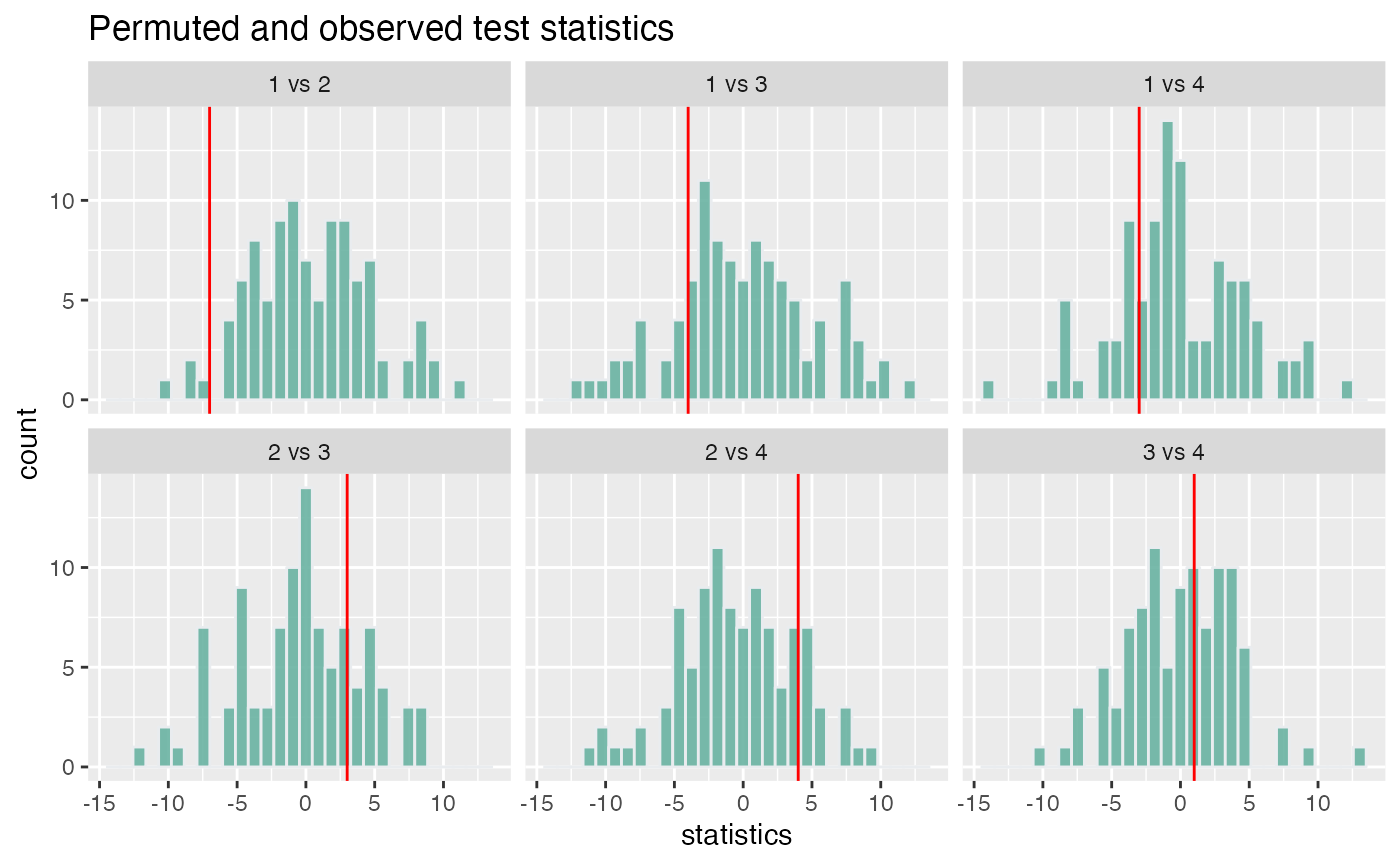The default of all pairwise comparisons.

perm5 = cluster_permute_test(aa80_chain\$TRB, cell_covariate_keys = 'ident', cell_stratify_keys = 'sample',
cell_label_key = 'aa80', n_perm = 100, statistic = compare_expanded_vec, contrasts = contrast_vec)

plot_permute_test(perm5)
#> stat_bin() using bins = 30. Pick better value with binwidth.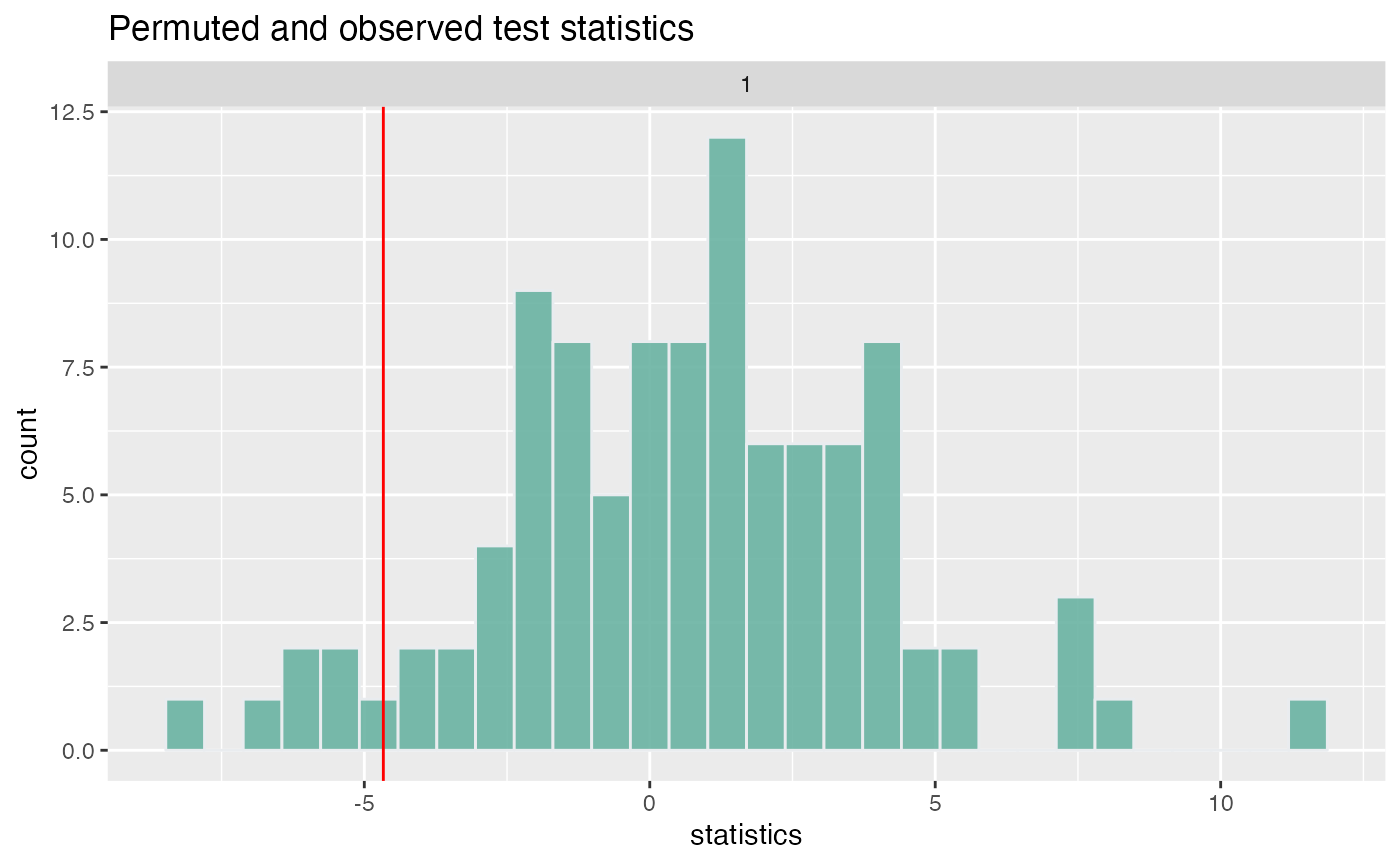tidy.PermuteTestList(perm5)
#> # A tibble: 100 × 7
#>    statistics observed expected p.value mc.se contrast_name contrast
#>         <dbl>    <dbl>    <dbl>   <dbl> <dbl> <chr>         <chr>
#>  1  -2.00e+ 0    -4.67    0.677    0.12 0.346 1             1, -0.3333333333333…
#>  2  -3   e+ 0    -4.67    0.677    0.12 0.346 1             1, -0.3333333333333…
#>  3  -2.00e+ 0    -4.67    0.677    0.12 0.346 1             1, -0.3333333333333…
#>  4   1.67e+ 0    -4.67    0.677    0.12 0.346 1             1, -0.3333333333333…
#>  5   1.67e+ 0    -4.67    0.677    0.12 0.346 1             1, -0.3333333333333…
#>  6  -8.33e+ 0    -4.67    0.677    0.12 0.346 1             1, -0.3333333333333…
#>  7  -7   e+ 0    -4.67    0.677    0.12 0.346 1             1, -0.3333333333333…
#>  8  -3.33e- 1    -4.67    0.677    0.12 0.346 1             1, -0.3333333333333…
#>  9  -2.67e+ 0    -4.67    0.677    0.12 0.346 1             1, -0.3333333333333…
#> 10   2.78e-16    -4.67    0.677    0.12 0.346 1             1, -0.3333333333333…
#> # … with 90 more rows

There is also a tidy method.

## Colophone

sessionInfo()
#> R version 4.1.2 (2021-11-01)
#> Platform: aarch64-apple-darwin20 (64-bit)
#> Running under: macOS Monterey 12.3.1
#>
#> Matrix products: default
#> BLAS:   /Library/Frameworks/R.framework/Versions/4.1-arm64/Resources/lib/libRblas.0.dylib
#> LAPACK: /Library/Frameworks/R.framework/Versions/4.1-arm64/Resources/lib/libRlapack.dylib
#>
#> locale:
#>  en_US.UTF-8/en_US.UTF-8/en_US.UTF-8/C/en_US.UTF-8/en_US.UTF-8
#>
#> attached base packages:
#>  stats     graphics  grDevices utils     datasets  methods   base
#>
#> other attached packages:
#>  ggdendro_0.1.23        purrr_0.3.4            stringr_1.4.0
#>  dplyr_1.0.8            CellaRepertorium_1.7.1 BiocStyle_2.22.0
#>
#> loaded via a namespace (and not attached):
#>   nlme_3.1-153           bitops_1.0-7           fs_1.5.2
#>   RColorBrewer_1.1-2     progress_1.2.2         rprojroot_2.0.2
#>   GenomeInfoDb_1.30.1    tools_4.1.2            backports_1.4.1
#>  bslib_0.3.1            utf8_1.2.2             R6_2.5.1
#>  DBI_1.1.2              BiocGenerics_0.40.0    colorspace_2.0-3
#>  withr_2.5.0            prettyunits_1.1.1      tidyselect_1.1.2
#>  compiler_4.1.2         textshaping_0.3.6      cli_3.2.0
#>  desc_1.4.1             labeling_0.4.2         bookdown_0.25
#>  sass_0.4.1             scales_1.1.1           pkgdown_2.0.2
#>  systemfonts_1.0.4      digest_0.6.29          minqa_1.2.4
#>  rmarkdown_2.13         XVector_0.34.0         pkgconfig_2.0.3
#>  htmltools_0.5.2        lme4_1.1-28            fastmap_1.1.0
#>  highr_0.9              rlang_1.0.2            rstudioapi_0.13
#>  jquerylib_0.1.4        farver_2.1.0           generics_0.1.2
#>  jsonlite_1.8.0         broom.mixed_0.2.7      RCurl_1.98-1.6
#>  magrittr_2.0.2         GenomeInfoDbData_1.2.7 Matrix_1.3-4
#>  Rcpp_1.0.8             munsell_0.5.0          S4Vectors_0.32.3
#>  fansi_1.0.2            lifecycle_1.0.1        stringi_1.7.6
#>  yaml_2.3.5             MASS_7.3-54            zlibbioc_1.40.0
#>  plyr_1.8.6             grid_4.1.2             forcats_0.5.1
#>  crayon_1.5.0           lattice_0.20-45        Biostrings_2.62.0
#>  cowplot_1.1.1          splines_4.1.2          hms_1.1.1
#>  knitr_1.37             pillar_1.7.0           boot_1.3-28
#>  reshape2_1.4.4         stats4_4.1.2           glue_1.6.2
#>  evaluate_0.15          BiocManager_1.30.16    vctrs_0.3.8
#>  nloptr_2.0.0           tzdb_0.2.0             gtable_0.3.0
#>  assertthat_0.2.1       cachem_1.0.6           xfun_0.30
#>  broom_0.7.12           ragg_1.2.2             tibble_3.1.6
#>  memoise_2.0.1          IRanges_2.28.0         ellipsis_0.3.2